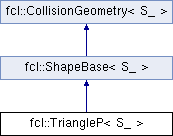FCL  0.6.0 Flexible Collision Library
fcl::TriangleP< S_ > Class Template Reference

Triangle stores the points instead of only indices of points. More...

`#include <triangle_p.h>`

Inheritance diagram for fcl::TriangleP< S_ >:## Public Types

using S = S_Public Types inherited from fcl::ShapeBase< S_ >
using S = S_

## Public Member Functions

TriangleP (const Vector3< S > &a, const Vector3< S > &b, const Vector3< S > &c)

void computeLocalAABB () override
virtual function of compute AABB<S> in local coordinate

NODE_TYPE getNodeType () const override
get the node type

std::vector< Vector3< S > > getBoundVertices (const Transform3< S > &tf) const
get the vertices of some convex shape which can bound this shape in a specific configurationPublic Member Functions inherited from fcl::ShapeBase< S_ >
OBJECT_TYPE getObjectType () const
Get object type: a geometric shape.Public Member Functions inherited from fcl::CollisionGeometry< S_ >
void * getUserData () const
get user data in geometry

void setUserData (void *data)
set user data in geometry

bool isOccupied () const
whether the object is completely occupied

bool isFree () const
whether the object is completely free

bool isUncertain () const
whether the object has some uncertainty

virtual Vector3< S_ > computeCOM () const
compute center of mass

virtual Matrix3< S_ > computeMomentofInertia () const
compute the inertia matrix, related to the origin

virtual S_ computeVolume () const
compute the volume

virtual Matrix3< S_ > computeMomentofInertiaRelatedToCOM () const
compute the inertia matrix, related to the com

## Public Attributes

Vector3< S > a

Vector3< S > b

Vector3< S > cPublic Attributes inherited from fcl::CollisionGeometry< S_ >
Vector3< S_ > aabb_center
AABB center in local coordinate.

AABB< S_ > aabb_local
AABB in local coordinate, used for tight AABB when only translation transform.

void * user_data
pointer to user defined data specific to this object

S_ cost_density
collision cost for unit volume

S_ threshold_occupied
threshold for occupied ( >= is occupied)

S_ threshold_free